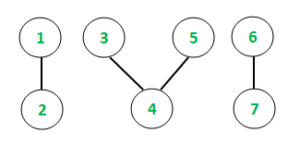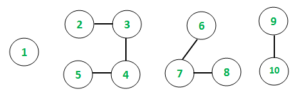# Maximum sum of values of nodes among all connected components of an undirected graph

Given an undirected graph with V vertices and E edges. Every node has been assigned a given value. The task is to find the connected chain with the maximum sum of of values among all the connected components in the graph.

Examples:

Input: V = 7, E = 4
Values = {10, 25, 5, 15, 5, 20, 0}Output : Max Sum value = 35
Explanation:
Component {1, 2} – Value {10, 25}: sumValue = 10 + 25 = 35
Component {3, 4, 5} – Value {5, 15, 5}: sumValue = 5 + 15 + 5 = 25
Component {6, 7} – Value {20, 0}: sumValue = 20 + 0 = 20
Max Sum value chain is {1, 2} with values {10, 25}, hence 35 is answer.

Input: V = 10, E = 6
Values = {5, 10, 15, 20, 25, 30, 35, 40, 45, 50}Output : Max Sum value = 105

## Recommended: Please try your approach on {IDE} first, before moving on to the solution.

Approach: The idea is to use the Depth First Search traversal method to keep a track of all the connected components. A temporary variable is used to sum up all the values of the individual values of the connected chains. At each traversal of a connected component, the heaviest value till now is compared with the current value and updated accordingly. After all connected components have been traversed, the maximum among all will the answer.

Below is the implementation of the above approach:

 `// C++ program to find Maximum sum of values ` `// of nodes among all connected ` `// components of an undirected graph ` `#include ` `using` `namespace` `std; ` ` `  `// Function to implement DFS ` `void` `depthFirst(``int` `v, vector<``int``> graph[], ` `                ``vector<``bool``>& visited, ` `                ``int``& sum, ` `                ``vector<``int``> values) ` `{ ` `    ``// Marking the visited vertex as true ` `    ``visited[v] = ``true``; ` ` `  `    ``// Updating the value of connection ` `    ``sum += values[v - 1]; ` ` `  `    ``// Traverse for all adjacent nodes ` `    ``for` `(``auto` `i : graph[v]) { ` ` `  `        ``if` `(visited[i] == ``false``) { ` ` `  `            ``// Recursive call to the DFS algorithm ` `            ``depthFirst(i, graph, visited, ` `                       ``sum, values); ` `        ``} ` `    ``} ` `} ` ` `  `void` `maximumSumOfValues(vector<``int``> graph[], ` `                        ``int` `vertices, vector<``int``> values) ` `{ ` `    ``// Initializing boolean array to mark visited vertices ` `    ``vector<``bool``> visited(values.size() + 1, ``false``); ` ` `  `    ``// maxChain stores the maximum chain size ` `    ``int` `maxValueSum = INT_MIN; ` ` `  `    ``// Following loop invokes DFS algorithm ` `    ``for` `(``int` `i = 1; i <= vertices; i++) { ` `        ``if` `(visited[i] == ``false``) { ` ` `  `            ``// Variable to hold temporary values ` `            ``int` `sum = 0; ` ` `  `            ``// DFS algorithm ` `            ``depthFirst(i, graph, visited, ` `                       ``sum, values); ` ` `  `            ``// Conditional to update max value ` `            ``if` `(sum > maxValueSum) { ` `                ``maxValueSum = sum; ` `            ``} ` `        ``} ` `    ``} ` ` `  `    ``// Printing the heaviest chain value ` `    ``cout << ``"Max Sum value = "``; ` `    ``cout << maxValueSum << ``"\n"``; ` `} ` ` `  `// Driver function to test above function ` `int` `main() ` `{ ` `    ``// Initializing graph in the form of adjacency list ` `    ``vector<``int``> graph; ` ` `  `    ``// Defining the number of edges and vertices ` `    ``int` `E = 4, V = 7; ` ` `  `    ``// Assigning the values for each ` `    ``// vertex of the undirected graph ` `    ``vector<``int``> values; ` `    ``values.push_back(10); ` `    ``values.push_back(25); ` `    ``values.push_back(5); ` `    ``values.push_back(15); ` `    ``values.push_back(5); ` `    ``values.push_back(20); ` `    ``values.push_back(0); ` ` `  `    ``// Constructing the undirected graph ` `    ``graph.push_back(2); ` `    ``graph.push_back(1); ` `    ``graph.push_back(4); ` `    ``graph.push_back(3); ` `    ``graph.push_back(5); ` `    ``graph.push_back(3); ` `    ``graph.push_back(7); ` `    ``graph.push_back(6); ` ` `  `    ``maximumSumOfValues(graph, V, values); ` `    ``return` `0; ` `} `

Output:

```Max Sum value = 35
```

Time Complexity: O(E + V)My Personal Notes arrow_drop_upCheck out this Author's contributed articles.

If you like GeeksforGeeks and would like to contribute, you can also write an article using contribute.geeksforgeeks.org or mail your article to contribute@geeksforgeeks.org. See your article appearing on the GeeksforGeeks main page and help other Geeks.

Please Improve this article if you find anything incorrect by clicking on the "Improve Article" button below.